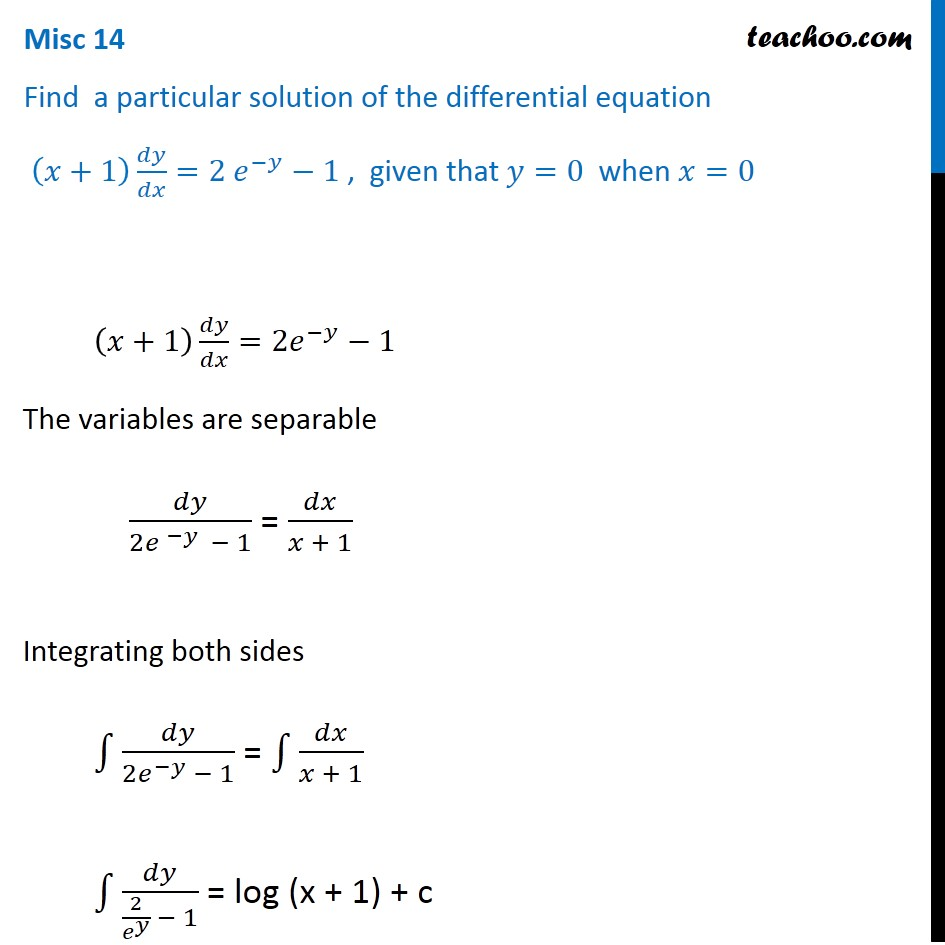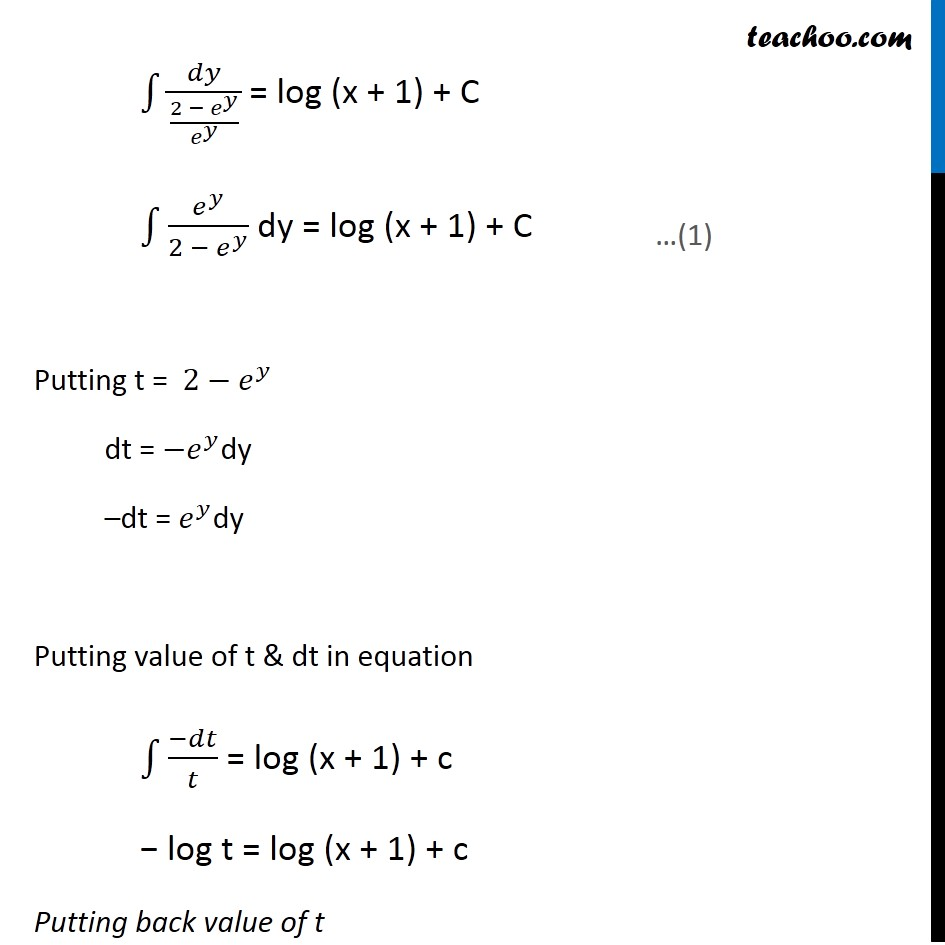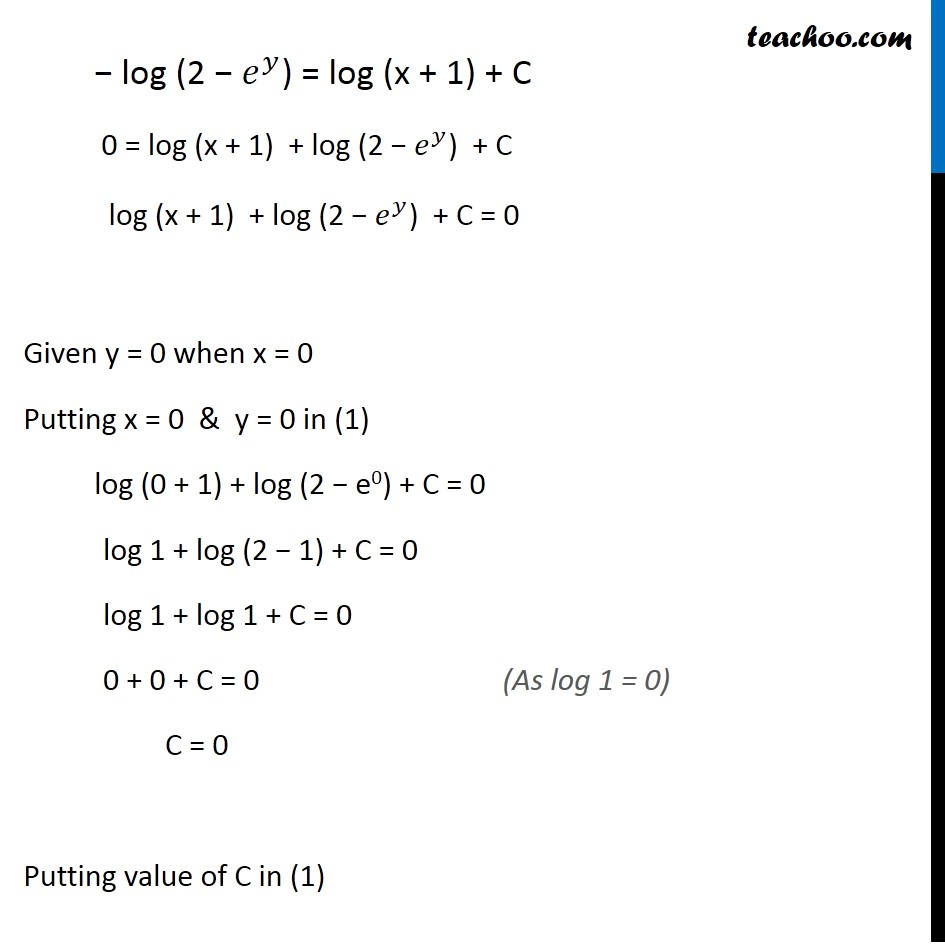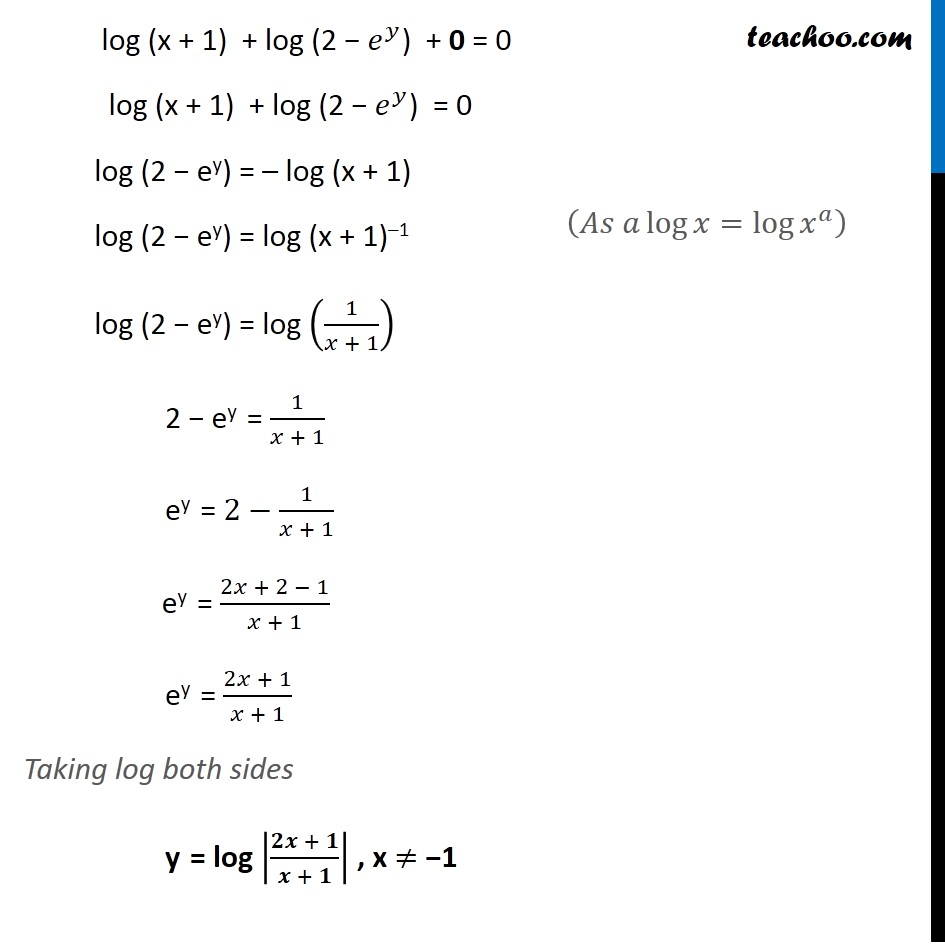Miscellaneous

Chapter 9 Class 12 Differential Equations
Serial order wiseGet live Maths 1-on-1 Classs - Class 6 to 12

### Transcript

Misc 14 Find a particular solution of the differential equation (𝑥+1) 𝑑𝑦/𝑑𝑥=2 𝑒^(−𝑦)−1 , given that 𝑦=0 when 𝑥=0 (𝑥+1) 𝑑𝑦/𝑑𝑥=2𝑒^(−𝑦)−1 The variables are separable 𝑑𝑦/(2𝑒^( −𝑦 ) − 1) = 𝑑𝑥/(𝑥 + 1) Integrating both sides ∫1▒𝑑𝑦/(2𝑒^(−𝑦) − 1) = ∫1▒𝑑𝑥/(𝑥 + 1) ∫1▒𝑑𝑦/(2/𝑒^𝑦 − 1) = log (x + 1) + c ∫1▒𝑑𝑦/((2 − 𝑒^𝑦)/𝑒^𝑦 ) = log (x + 1) + C ∫1▒𝑒^𝑦/(2 −〖 𝑒〗^𝑦 ) dy = log (x + 1) + C Putting t = 2−𝑒^𝑦 dt = −𝑒^𝑦dy –dt = 𝑒^𝑦dy Putting value of t & dt in equation ∫1▒(−𝑑𝑡)/𝑡 = log (x + 1) + c − log t = log (x + 1) + c Putting back value of t …(1) − log (2 − 𝑒^𝑦) = log (x + 1) + C 0 = log (x + 1) + log (2 − 𝑒^𝑦) + C log (x + 1) + log (2 − 𝑒^𝑦) + C = 0 Given y = 0 when x = 0 Putting x = 0 & y = 0 in (1) log (0 + 1) + log (2 − e0) + C = 0 log 1 + log (2 − 1) + C = 0 log 1 + log 1 + C = 0 0 + 0 + C = 0 C = 0 Putting value of C in (1) (As log 1 = 0) log (x + 1) + log (2 − 𝑒^𝑦) + 0 = 0 log (x + 1) + log (2 − 𝑒^𝑦) = 0 log (2 − ey) = – log (x + 1) log (2 − ey) = log (x + 1)–1 log (2 − ey) = log (1/(𝑥 + 1)) 2 − ey = 1/(𝑥 + 1) ey = 2−1/(𝑥 + 1) ey = (2𝑥 + 2 − 1)/(𝑥 + 1) ey = (2𝑥 + 1)/(𝑥 + 1) Taking log both sides y = log |(𝟐𝒙 + 𝟏)/(𝒙 + 𝟏)| , x ≠ −1 (𝐴𝑠 𝑎 log⁡〖𝑥=log⁡〖𝑥^𝑎 〗 〗 )